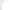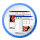Look RS232 > RS232C HardwareLine Control Register (LCR)The Line Control register sets the general connection parameters.

Bits 0 and 1 define the word length (number of data bits). Legitimate values are given in the table. 8 bit length is usually used today.

Bit 2 sets the length of the stop bits. Setting this bit into '0' provides length setting equal to 1 bit, if the bit is set into '1', the length of stop bits is 2 (if the length of data bits is 6,7 or 8 bits) or 1.5 bits (if the length of data bits is 6,7 or 8 bits).

Bits 3, 4 and 5 define parity mode. When defining these 3 bits you can set any parity mode. If the third bit is '0', there is no parity check, if this bit is set to '1', parity check is carried out, and the check mode depends on the values set in bits 4 and 5. Possible variants are given in the table.

Bit 6 sets break enable. When active, the TD line goes into "Spacing" state which causes a break in the receiving UART. Setting this bit to '0' disables the Break.

Bit 7 is the Divisor Latch Access Bit (DLAB). We have already mentioned this bit.

 Bit 7 1 Divisor Latch Access Bit 0 Access to Receiver buffer, Transmitter buffer & Interrupt Enable Register Bit 6 Set Break Enable Bits 3, 4 And 5 Bit 5 Bit 4 Bit 3 Parity Select X X 0 No Parity 0 0 1 Odd Parity 0 1 1 Even Parity 1 0 1 High Parity (Sticky) 1 1 1 Low Parity (Sticky) Bit 2 Length of Stop Bit 0 One Stop Bit 1 2 Stop bits for words of length 6,7 or 8 bits or 1.5 Stop Bits for Word lengths of 5 bits. Bits 0 And 1 Bit 1 Bit 0 Word Length 0 0 5 Bits 0 1 6 Bits 1 0 7 Bits 1 1 8 Bits
Line Control Register